# Probabilistic Programming 4: Gaussian Dynamical System¶

## Variational inference¶

### Preliminaries¶

• Goal
• Learn to apply ForneyLab to a dynamical system.
• Materials
• Mandatory
• These lecture notes.
• Optional
In [ ]:
using Pkg
Pkg.activate("workspace")
Pkg.instantiate()

using Logging; disable_logging(LogLevel(0))
using LinearAlgebra
using LaTeXStrings
using ForneyLab
using Plots
pyplot()
include("../scripts/pp-4.jl");


## Data generation¶

In this notebook, we will be considering continuous state-space models. We will focus on the simplest form: the Gaussian dynamical system:

\begin{align} x_k =&\ A x_{k-1} + \epsilon_{k} \\ y_k =&\ B x_k + \eta_k \, , \end{align}

where $x_k \in \mathbb{R}^{N}$ is the state, $y_k \in \mathbb{R}^{M}$ is the measurement. Process noise and measurement noise are assumed to be zero mean Gaussian with covariance matrices $Q$ and $R$ respectively.

We'll generate the data in the same manner as the HMM setting. We start with an initial state $x_0$, apply the state transition $Ax_0$, and add Gaussian noise $\epsilon_1$. We then take the current state $x_1$, apply the emission transformation $B x_1$ and add Gaussian noise again $\eta_1$. This is repeated until we've reached $T$ observations.

In :
# Dimensionalities
N = 2
M = 2

# Length of time-series
T = 50

# Lenght of time step
Δt = 1.0

# Transition matrix of latent variables
transition = [1.0 Δt;
0.0 1.0]

# Emission matrix for observed variables
emission = [1.0 0.;0. 1.0]

# Process noise (latent variables)
process_noise = [0.2*Δt 0.;0. 0.1*Δt]

# Measurement noise (observations)
measurement_noise = [0.1 0.;0. 0.1]

# Preallocate data arrays
states = zeros(N, T)
observations = zeros(M, T)

# Initial state
state_0 = [0., 0.]

# Keep previous state in memory
state_tmin = state_0

# Generate data for entire time-series
for t = 1:T

# Transition from previous state
states[:,t] = sample(ProbabilityDistribution(Multivariate, GaussianMeanVariance, m=transition * state_tmin, v=process_noise))

# Emission of current state
observations[:,t] = sample(ProbabilityDistribution(Multivariate, GaussianMeanVariance, m=emission * states[:,t], v=measurement_noise))

# Update previous state
state_tmin = states[:,t]

end

# Visualization of states
plot(states[1,:], states[2,:], color="red", label="states", grid=false, xlabel="position x-coordinate", ylabel="position y-coordinate")
scatter!(observations[1,:], observations[2,:], color="blue", label="observations")

Out: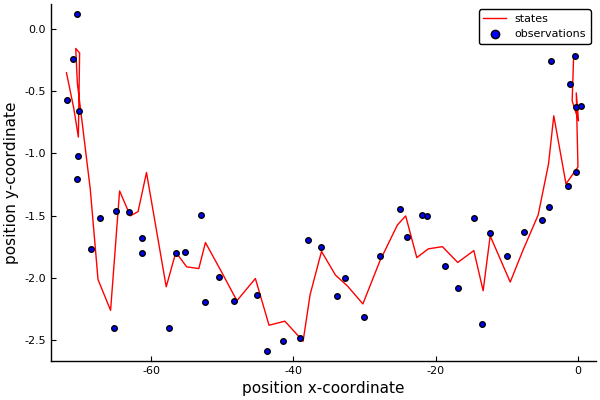Since we don't have a time-axis anymore, it is hard to see how the process evolves. Animating the path is helpful.

In :
"Animation of cart's path"

# Plot initial state
Plots.plot([states[1,1]], [states[2,1]],
color="red",
label="states",
grid=false,
xlabel="position x-coordinate",
ylabel="position y-coordinate",
xlims=[-60,60],
ylims=[-10,10])

# Plot initial observation
scatter!([observations[1,1]], [observations[2,1]], color="blue", label="observations")

anim = @animate for t = 2:T

title!("t = "*string(t))

# Plot new state
plot!([states[1,t-1:t]], [states[2,t-1:t]], color="red", label="")

# Plot new observation
scatter!([observations[1,t]], [observations[2,t]], color="blue", label="")

end

gif(anim, "visualizations/example-GDS.gif", fps = 3)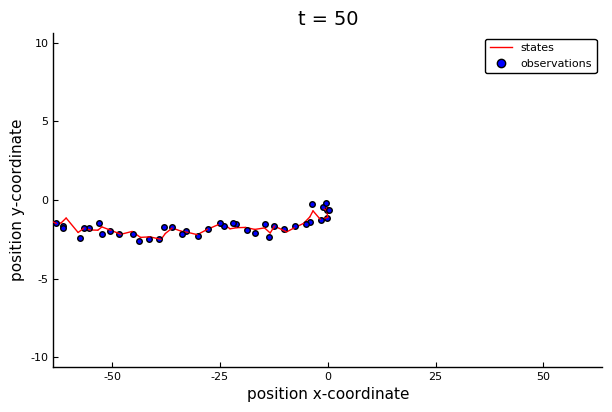Out: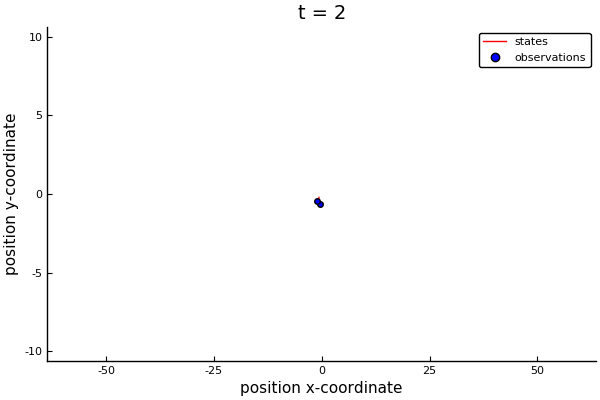## Model specification¶

The Bayesian formulation of the LGDS state-space model takes the following form:

\begin{align} p(x_k \mid x_{k-1}) =&\ \mathcal{N}(x_k \mid A x_{k-1}, Q)\\ p(y_k \mid x_k) =&\ \mathcal{N}(y_k \mid B x_k, R) \, . \end{align}

We can easily specify the states and observations as multivariate Gaussian distributions. If we do not know the process and measurement noise matrices, we can add Wishart priors to them. Similarly, if we don't know the transition and emission matrices, we can priors for them as well. Since these can take on negative values, we would specify them as multivariate Gaussians;

\begin{align} p(Q) \sim&\ \mathcal{W}(V_Q, n_Q) \\ p(R) \sim&\ \mathcal{W}(V_R, n_R) \\ p(A) \sim&\ \mathcal{N}(m_A, S_A) \\ p(B) \sim&\ \mathcal{N}(m_B, S_B) \, . \end{align}

ForneyLab is designed for dynamical systems and excels when using Gaussians. It can do inference in a recursive, time-slice-based manner. That is, it performs a series of variational message passing iterations in each time-slice, moving forward through time.

### Model 1: state estimation¶

We will stick to state estimation for now. We will dynamically "fix" the previous states and observations, allowing us to focus the variational message passing procedure to the "current" state and observation in the time-slice.

In :
# Initialize a graph
graph1 = FactorGraph()

# Preallocate variable arrays
x = Vector{Variable}(undef, T)
y = Vector{Variable}(undef, T)

# Prior for initial state
@RV x_0 ~ GaussianMeanPrecision([0., 0.], [1. 0.;0. 1.])

# Keep previous state in memory
x_tmin = x_0

# Iterate over time
for t = 1:T

# State transition
@RV ϵ_t ~ GaussianMeanVariance([0., 0.], process_noise)
@RV x[t] = transition * x_tmin + ϵ_t
x[t].id = :x_*t

# State transition
@RV η_t ~ GaussianMeanVariance([0., 0.], measurement_noise)
@RV y[t] = emission * x[t] + η_t
y[t].id = :x_*t

# Tell FL that y is observed
placeholder(y[t], :y, index=t, dims=(2,))

# Update previous state
x_tmin = x[t]
end


We'll now construct the algorithm and infer results.

In :
# Number of optimization iterations
num_iterations = 10

# Define recognition distribution
q = RecognitionFactorization(x, ids=[:X])

# Generate variational algorithm
algo = variationalAlgorithm(q)

eval(Meta.parse(algo))

# Initialize recognition distribution marginals
marginals = Dict{Symbol, ProbabilityDistribution}()
for t = 1:T
marginals[:x_*t] = vague(ForneyLab.GaussianMeanVariance, 2)
end

# Initialize data
data = Dict(:y => [observations[:,t] for t = 1:T])

# Run algorithm
for i = 1:num_iterations

# Update states
stepX!(data, marginals)

end;


Let's check whether everything went ok. We'll visualize the state estimations.

In :
# Preallocation
states_hat = zeros(2,T)
states_sem = zeros(2,T)

for t = 1:T

# State estimates
states_hat[:,t] = mean(marginals[:x_*t])

# Variance of the state estimates
states_sem[:,t] = sqrt.(var(marginals[:x_*t]))
end

# Visualization
plot(states[1,:], states[2,:], color="red", label="states", grid=false)
plot!(states_hat[1,:], states_hat[2,:], ribbon=[states_sem, states_sem], fillalpha=0.2, color="green", label="inferred")
scatter!(observations[1,:], observations[2,:], color="blue", label="observations")

Out: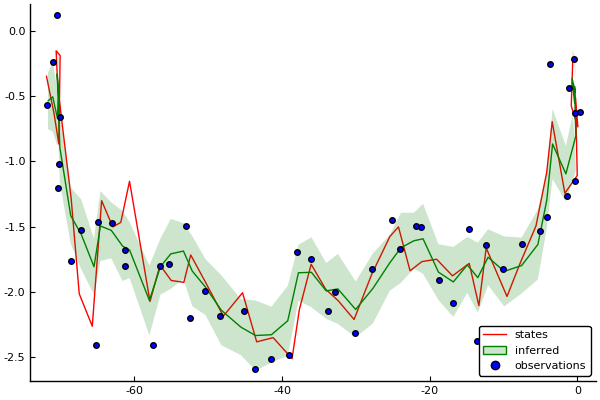We're going to inspect some messages. Let's open up the algorithm. Let's look up the marginal for $x_1$. It will be the multiplication of two messages, one consisting of the state transition prediction and the other consisting of the measurement likelihood.

In :
# Find index of marginal computation in algorithm string
ix = findfirst("marginals[:x_1]", algo)

# Print line at index
println(algo[ix:ix[end]+42])

marginals[:x_1] = messages.dist * messages.dist


We can re-run the inference algorithm such that we get an array of all messages in the graph.

In :
# Re-initialize recognition distribution marginals
marginals = Dict{Symbol, ProbabilityDistribution}()
for t = 1:T
marginals[:x_*t] = vague(ForneyLab.GaussianMeanVariance, 2)
end

# Initialize messages
messages = Vector{Message}(undef, 698)

# Re-run inference algorithm now including the message array
for i = 1:num_iterations

# Update states
stepX!(data, marginals, messages)

end;


Let's visualize the two messages and the resulting state marginal.

In :
# Collect state transition prediction
m_statepred = convert(ProbabilityDistribution{Multivariate, GaussianMeanVariance}, messages.dist)

# Collect measurement likelihood
m_likelihood = convert(ProbabilityDistribution{Multivariate, GaussianMeanVariance}, messages.dist)

# Collect corrected prediction
state_marginal = convert(ProbabilityDistribution{Multivariate, GaussianMeanVariance}, marginals[:x_1])

# # Extract x-coordinates
m_statepred_x = ProbabilityDistribution(Univariate, GaussianMeanVariance, m=m_statepred.params[:m], v=m_statepred.params[:v][1,1])
m_likelihood_x = ProbabilityDistribution(Univariate, GaussianMeanVariance, m=m_likelihood.params[:m], v=m_likelihood.params[:v][1,1])
state_marginal_x = ProbabilityDistribution(Univariate, GaussianMeanVariance, m=state_marginal.params[:m], v=state_marginal.params[:v][1,1])

# Plot of the prediction, noisy measurement, and corrected prediction for x-coordinate
plot_messages(m_statepred_x, m_likelihood_x, state_marginal_x)
title!(L"Factors contributing to marginal of state $x_1$")

Out: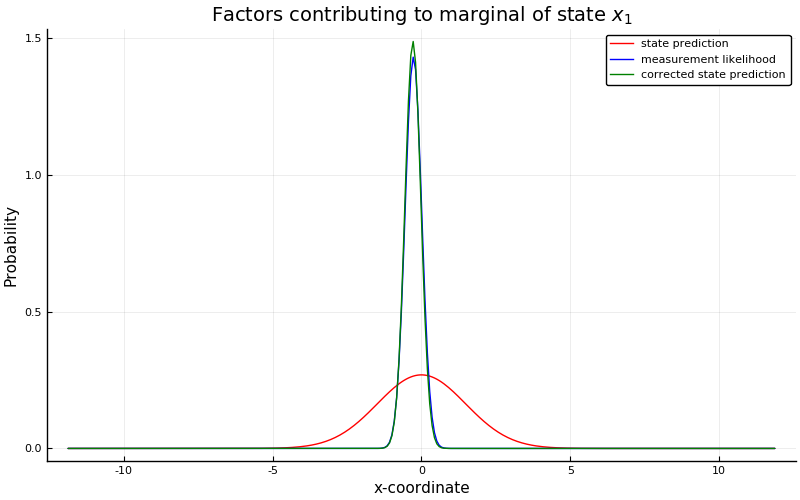The state marginal is dominated by the observation, because the state prediction is based purely on a prior distribution. If we now look at the situation for $x_2$, things should be different.

In :
# Collect state transition prediction
m_statepred = convert(ProbabilityDistribution{Multivariate, GaussianMeanVariance}, messages.dist)

# Collect measurement likelihood
m_likelihood = convert(ProbabilityDistribution{Multivariate, GaussianMeanVariance}, messages.dist)

# Collect corrected prediction
state_marginal = convert(ProbabilityDistribution{Multivariate, GaussianMeanVariance}, marginals[:x_2])

# # Extract x-coordinates
m_statepred_x = ProbabilityDistribution(Univariate, GaussianMeanVariance, m=m_statepred.params[:m], v=m_statepred.params[:v][1,1])
m_likelihood_x = ProbabilityDistribution(Univariate, GaussianMeanVariance, m=m_likelihood.params[:m], v=m_likelihood.params[:v][1,1])
state_marginal_x = ProbabilityDistribution(Univariate, GaussianMeanVariance, m=state_marginal.params[:m], v=state_marginal.params[:v][1,1])

# Plot of the prediction, noisy measurement, and corrected prediction for x-coordinate
plot_messages(m_statepred_x, m_likelihood_x, state_marginal_x)
title!(L"Factors contributing to marginal of state $x_2$")

Out: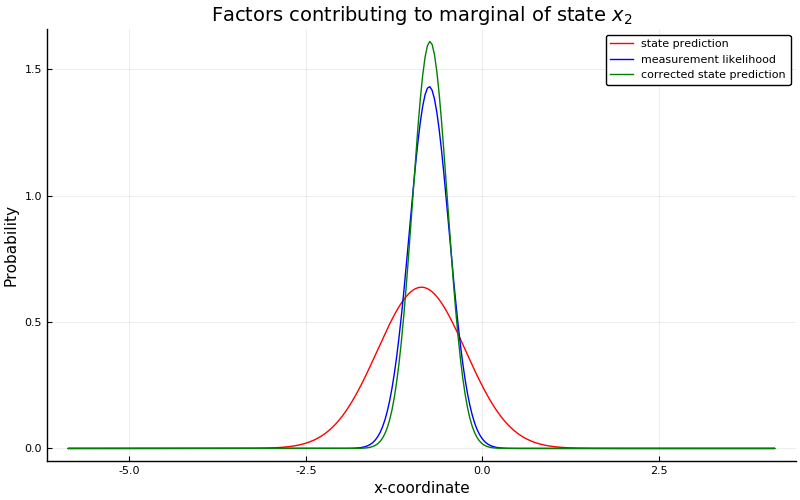Now, the state prediction has more influence on the actual state marginal.

### Model 2: estimate process noise¶

Weĺl now expand on the model. What if we don't know process noise? Can we estimate it simultaneously?

In :
# Initialize a graph
graph2 = FactorGraph()

# Preallocate variable arrays
x = Vector{Variable}(undef, T)
y = Vector{Variable}(undef, T)

# Prior for process noise
@RV Q ~ Wishart([1. 0.;0. 1.], 2, id=:Q)

# Prior for initial state
@RV x_0 ~ GaussianMeanPrecision([0., 0.], [1. 0.;0. 1.])

# Keep previous state in memory
x_tmin = x_0

# Iterate over time
for t = 1:T

# State transition
@RV ϵ_t ~ GaussianMeanPrecision([0., 0.], Q, id=:ϵ_*t)
@RV x[t] = transition * x_tmin + ϵ_t
x[t].id = :x_*t

# State transition
@RV η_t ~ GaussianMeanVariance([0., 0.], measurement_noise, id=:η_*t)
@RV y[t] = emission * x[t] + η_t
y[t].id = :y_*t

# Tell FL that y is observed
placeholder(y[t], :y, index=t, dims=(2,))

# Update previous state
x_tmin = x[t]
end


We'll need to add a recognition factor for the process noise covariance matrix $Q$.

In :
# Define recognition distribution
q = RecognitionFactorization(x, Q, ids=[:X, :Q])

# Generate variational algorithm
algo = variationalAlgorithm(q)

eval(Meta.parse(algo))

# Initialize recognition distribution marginals
marginals = Dict{Symbol, ProbabilityDistribution}()
marginals[:Q] = vague(ForneyLab.Wishart, 2)
for t = 1:T
marginals[:x_*t] = vague(ForneyLab.GaussianMeanVariance, 2)
end

# Initialize data
data = Dict(:y => [observations[:,t] for t = 1:T])

# Run algorithm
for i = 1:num_iterations

# Update states
stepX!(data, marginals)

# Update process noise estimate
stepQ!(data, marginals)

end;

In :
# Preallocation
states_hat = zeros(2,T)
states_sem = zeros(2,T)

for t = 1:T

# State estimates
states_hat[:,t] = mean(marginals[:x_*t])

# Variance of the state estimates
states_sem[:,t] = sqrt.(var(marginals[:x_*t]))
end

# Visualization
Plots.plot(states[1,:], states[2,:], color="red", label="states", grid=false)
plot!(states_hat[1,:], states_hat[2,:], ribbon=[states_sem, states_sem], fillalpha=0.2, color="green", label="inferred")
scatter!(observations[1,:], observations[2,:], color="blue", label="observations")

Out: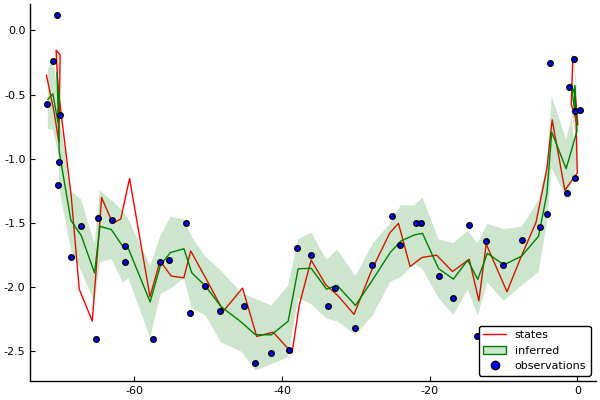In :
# Estimated process noise
pnoise_estimate = inv(mean(marginals[:Q]))

# Visualization
l = @layout [a b]
h1 = Plots.bar(0:4, reshape(process_noise, (4,)), label="true", ylims=[0., 1.])
h2 = Plots.bar(reshape(pnoise_estimate, (4,)), label="estimated", ylims=[0., 1.])
Plots.plot(h1, h2, layout=l, size=(1000,500))

Out: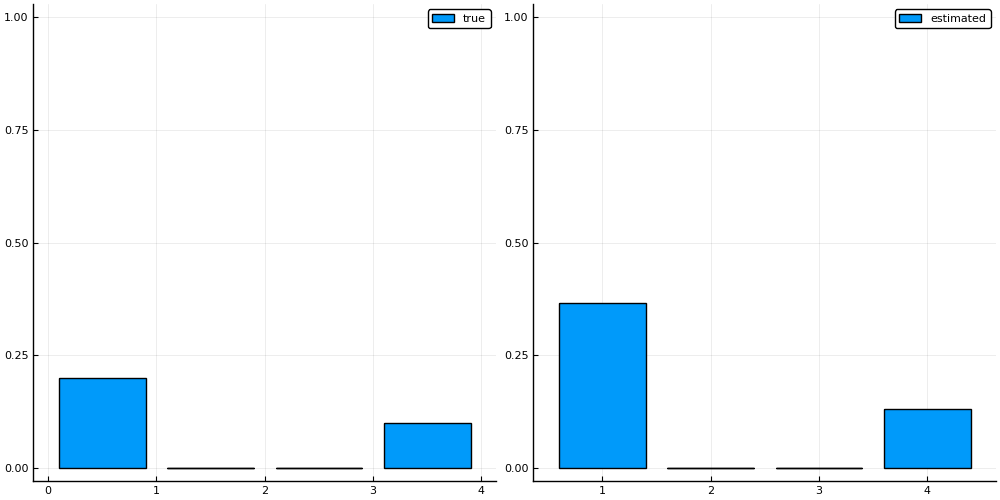### Optional assignment: transition matrix estimation¶

The transition matrix can be estimated in a similar fashion as the process noise matrix, except that it does not need to be positive semi-definite. Can you construct a model with states, process noise and the transition matrix as free variables?

In :
### Your code here